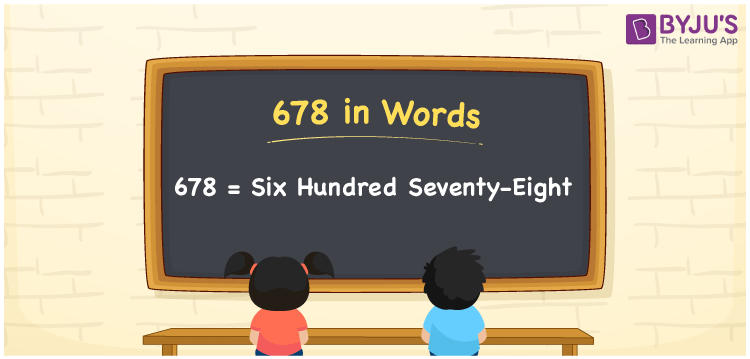# 678 in words

678 in words is written as Six Hundred and Seventy Eight. 678 represents the count or value. The article on Counting Numbers can give you an idea about count or counting. The number 678 is used in expressions that relate to money, distance, length, year and others. Let us consider an example for 678. ”Can you lend me Six Hundred and Seventy Eight rupees?”

 678 in words Six Hundred and Seventy Eight Six Hundred and Seventy Eight in Numbers 678

## 678 in English Words## How to Write 678 in Words?

We can convert 678 to words using a place value chart. The number 678 has 3 digits, so let’s make a chart that shows the place value up to 3 digits.

 Hundreds Tens Ones 6 7 8

Thus, we can write the expanded form as:

= 6 × Hundred + 7 × Ten + 8 × One

= 6 × 100 + 7 × 10 + 8 × 1

= 678

= Six Hundred and Seventy Eight.

678 is the natural number that is succeeded by 677 and preceded by 679.

678 in words – Six Hundred and Seventy Eight.

Is 678 an odd number? – No.

Is 678 an even number? – Yes.

Is 678 a perfect square number? – No.

Is 678 a perfect cube number? – No.

Is 678 a prime number? – No.

Is 678 a composite number? – Yes.

## Solved Example

1. Write the number 678 in expanded form

Solution: 6 × 100 + 7 × 10 + 8 × 1

We can write 678 = 600 + 70 + 8

= 6 × 100 + 7 × 10 + 8 × 1

## Frequently Asked Questions on 678 in words

Q1

### How to write 678 in words?

678 in words is written as Six Hundred and Seventy Eight.
Q2

### Is 678 a perfect square number?

No. 678 is not a perfect square number.
Q3

### Is 678 a prime number?

No. 678 is not a prime number.